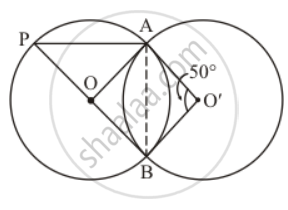Advertisement Remove all ads

# In the Given Figure, Two Congruent Circles with Centres O and O' Intersect at a and B. If ∠Aob = 50°, Then Find ∠Apb. - Mathematics

Answer in Brief

In the given figure, two congruent circles with centres O and O' intersect at A and B. If ∠AOB = 50°, then find ∠APB.Advertisement Remove all ads

#### Solution

Since both the circles are congruent, they will have equal radii. Let their radii be ‘r’.

So, from the given figure we have,

OA = OB = O'A = O'B = rNow, since all the sides of the quadrilateral OBO’A are equal it has to be a rhombus.

One of the properties of a rhombus is that the opposite angles are equal to each other.

So, since it is given thatangle AO'B  = 50° , we can say that the angle opposite it, that is to say that angle AOB  should also have the same value.

Hence we get  angle AOB  = 50°

Now, consider the first circle with the centre ‘O’ alone. ‘AB’ forms a chord and it subtends an angle of 50° with its centre, that is .angle AOB  = 50°

A property of a circle is that the angle subtended by an arc at the centre of the circle is double the angle subtended by the arc in the remaining part of the circle.

This means that,

angle APB = (angleAOB)/2

=(50°)/2

= 25°

Hence the measure of angleAPB   is  25°

Is there an error in this question or solution?
Advertisement Remove all ads

#### APPEARS IN

RD Sharma Mathematics for Class 9
Chapter 15 Circles
Q 2 | Page 108
Advertisement Remove all ads

#### Video TutorialsVIEW ALL 

Advertisement Remove all ads
Share
Notifications

View all notifications

Forgot password?
Course